﻿

### Existence of limits of analytic one-parameter semigroups of copulas

#### Abstract

A 2-copula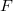$F$ is idempotent if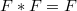$F*F=F$.  Here$*$ denotes the product      defined in .  An idempotent copula$F$ is said to be a unit      for a 2-copula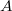$A$ if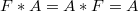$F*A=A*F=A$.  An idempotent copula is said to      annihilate a 2-copula$A$ if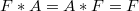$F*A=A*F=F$.
If$F$ is a unit for$A$ and$s$ is a non-negative real number, define
For any copula$A$ and any idempotent copula$F$ which is a unit for$A$, the set
is a semigroup of copulas under the$*$ operation, which is homomorphic to the semigroup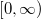$[0,\infty )$ under addition.  We call this set an analyticone-parameter semigroup of copulas.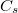$C_s$ can be defined also for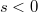$s<0$, and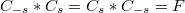$C_{-s}*C_s=C_s*C_{-s}=F$, but in general$C_s$ is not a copula for$s<0$.
We show that for any such analytic one-parameter semigroup, the limit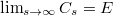$\lim_{s\to \infty}C_s=E$ exists.  We show also that the limit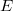$E$ has the followingproperties:
(i)$E$ is idempotent.
(ii)$E$ annihilates$A$,$F$ and$C_s$.
(iii)$E$ is the greatest annihilator of$A$ and of$C_s$,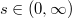$s\in (0,\infty )$.
\noindent It is also true that$F$ is the least unit for$C_s$,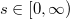$s\in [0,\infty)$.  We give a geometrical interpretation of this result, and we comment on theuse of analytic semigroups to construct Markov processes with continuousparameter.

DOI Code: 10.1285/i15900932v30n2p1

Keywords: copula; idempotent; star product

Full Text: PDF